SVG Guide# SVG Guide

## Overview

The user interface in a Fitbit application is described in SVG files, which is based upon the internet standard for scalable vector graphics.

``````<svg>
<image href="my-image.jpg" />
<text fill="red" font-family="System-Regular">Hello World!</text>
</svg>
``````

Fitbit OS projects typically contain two SVG files by default, but additional files can be added and imported, or used within the document view stack.

In Fitbit OS 5, the default SVG files are `index.view` which contains the default view for your app or clock face, and `widget.defs` which contains default imports and symbol definitions.

Note: In Fitbit OS 4, the default files are `index.gui` and `widgets.gui`.

## Coordinates and Encapsulation

There are a few basic principles of SVG that are important to understand.

First, the `x` and `y` coordinates are always expressed from the top-left corner of the screen. For example, to display a French flag:

``````<svg>
<rect x="0"   y="0" width="112" height="100%" fill="blue" />
<rect x="112" y="0" width="112" height="100%" fill="white" />
<rect x="224" y="0" width="112" height="100%" fill="red" />
</svg>
``````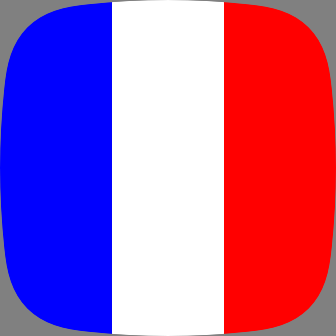Another important concept is the use of percentage in place of `x`, `y`, `width` or `height`.

``````<svg>
<rect x="0"   y="0" width="33%" height="100%" fill="blue" />
<rect x="33%" y="0" width="34%" height="100%" fill="white" />
<rect x="67%" y="0" width="33%" height="100%" fill="red" />
</svg>
``````

You can also combine percentages and pixels in the same value. For example `30%+15` or `30%-15`. These calculated values cannot contain spaces.

Some elements create a new coordinate context and you can encapsulate other elements in them. The most generic element that creates a new coordinate system is `<svg>`.

When inserted into such an element, the `x` and `y` coordinates of the child element are relative to the parent element. Percentages will also be relative to the parent.

``````<svg>
<svg x="10%" y="10%" width="80%" height="80%" fill="black">
<rect x="0"   y="0" width="33%" height="100%" fill="blue" />
<rect x="33%" y="0" width="34%" height="100%" fill="white" />
<rect x="67%" y="0" width="33%" height="100%" fill="red" />
</svg>
</svg>
``````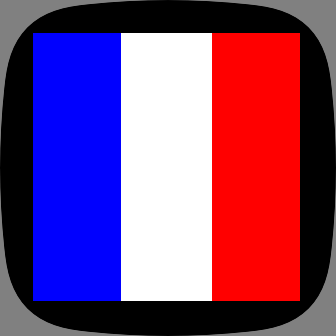## Lines

The `<line>` element draws a line between two pairs of coordinates.

• `x1`, `y1`: the start point of the line.
• `x2`, `y2`: the end point of the line.
• `fill`: specifies the line fill color.
• `stroke-width`: the thickness of the line. Maximum 32.
• `stroke-linecap`: `round` or `butt`, defaults to `round`, specifies if the end caps of lines should be rounded or squared.
• `opacity`: specifies the opacity of the line (between `0` and `1`).
• `visibility`: specifies if the line should be `visible` or `hidden`.
• `display`: `none`, `inline` or `inherit`.

The following example draws a simple diagonal line across the screen.

``````<svg viewport-fill="yellow">
<line x1="10" y1="10" x2="100%-10" y2="100%-10" fill="red" stroke-width="2" />
</svg>
``````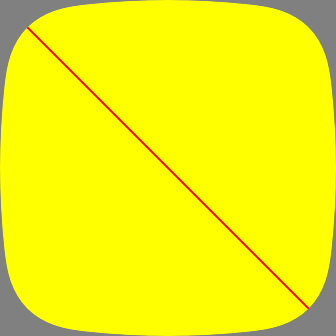## Rectangles

The `<rect>` element allows you to draw rectangles on the screen.

Supported attributes:

• `x` and `y`: position of the left, top-most corner.
• `width` and `height`: specify the rectangle's size.
• `fill`: specifies the rectangle fill color.
• `opacity`: specifies the opacity of the rectangle (between `0` and `1`).
• `visibility`: specifies if the rectangle should be `visible` or `hidden`.
• `display`: `none`, `inline` or `inherit`.
``````<svg viewport-fill="red">
<rect x="10%" y="10%" width="80%" height="80%" fill="blue" />
</svg>
``````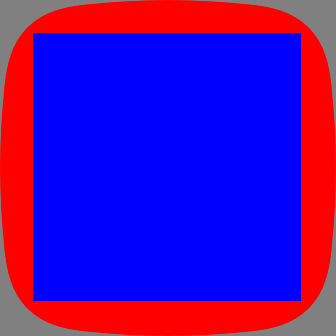## Circles

Attributes

• `cx`, `cy`: coordinates of the center `x` and center `y` of the circle.
• `r`: radius of the circle.
``````<svg viewport-fill="lightblue">
<circle cx="50%" cy="50%" r="80" fill="red" />
</svg>
``````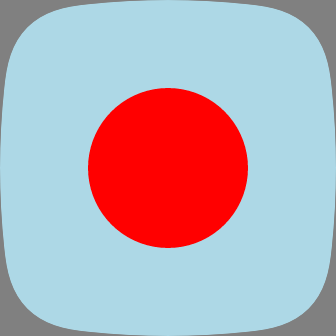## Images

You can display images in your application in either PNG or JPEG formats. PNG format is preferable as it is compiled into a hardware accelerated TXI format during compilation. JPEG files are smaller, but take longer to render. Note that Progressive JPEG files are not supported.

Attributes

• `x`, `y`: position of the left, top-most corner.
• `width`, `height`: specify the size of the image.

You must specify the size of the image. If you use a percentage, it will be relative to the parent container.

``````<svg viewport-fill="gray">
<image x="68" y="68" width="200" height="200" href="icon.png" />
</svg>
``````## Text

A single line of text.

Attributes

• `x`, `y`: coordinates of the anchor point.
• `fill`: color of the text.
• `text-anchor`: can be `start`, `middle`, `end`.
• `text-buffer`: holds the text string.
• `text-length`: specifies the number of characters to reserve for the text string. Will be rounded up to a multiple of 4.
• `letter-spacing`: in pixels.
• `text-overflow`: can be `clip` or `ellipsis`.
• `font-family`: specifies the font to use.
• `font-size`: specifies the font size in pixels.
• `font-weight`: specifies the character outline thickness (`light`, `regular`, or `bold`).
• `text-decoration`: specifies the if the text will be underlined or not.
``````<svg viewport-fill="yellow">
<text x="50%" y="50%+15"
font-family="System-Regular" fill="black"
font-size="30" font-weight="bold"
text-anchor="middle">Demo Text</text>
</svg>
``````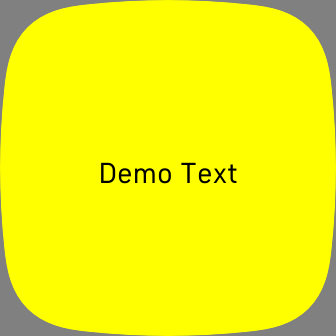When placing `<text>` elements, you are specifying the `x` and `y` coordinate of the anchor point. By default the anchor is the left side of the text baseline.

The baseline is at the bottom of the text. This means that a text element, placed at `0,0` will not be visible on screen because the text will be drawn above the baseline.

## Textarea

While `<text>` should be used for a single line of text, the `<textarea>` element displays text over multiple lines.

Attributes

• `x`, `y`: coordinates of the anchor point.
• `width` : specifies the maximum width of the display area.
• `height` : specifies the maximum height of the text, and the default is derived from the font file
• `fill`: color of the text.
• `text-anchor`: can be `start`, `middle`, `end`.
• `text-buffer`: holds the text string.
• `text-length`: specifies the number of characters to reserve for the text string. Will be rounded up to a multiple of 4.
• `letter-spacing`: in pixels.
• `text-overflow`: can be `clip` or `ellipsis`.
• `font-family`: specifies the font to use.
• `font-size`: specifies the font size in pixels.
• `font-weight`: specifies the character outline thickness (`light`, `regular`, or `bold`).
• `text-decoration`: specifies the if the text will be underlined or not.
• `display-align`: specifies the vertical alignment (`before`, `center`, `after`).
• `line-increment`: specifies the line, or row height.
• `rows`: constrains the number of rows.
``````<svg viewport-fill="yellow">
<textarea x="25" y="50" width="100%-50"
font-family="System-Regular" fill="black" font-size="24">Lorem ipsum dolor
sit amet, consectetur adipiscing elit. Hoc Hieronymus summum bonum esse
dixit. Cui Tubuli nomen odio non est? Teneo, inquit, finem illi videri nihil
dolere.</textarea>
</svg>
``````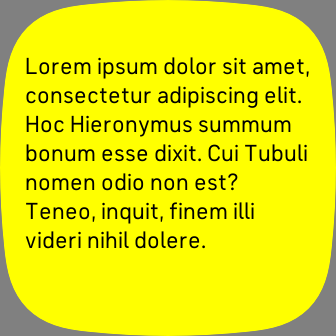When placing `<textarea>` elements, you are specifying the `x` and `y` coordinate of the top left anchor point. This is not the baseline coordinate.

Text will automatically wrap, or you can add a carriage return within the text to add a line break. If you want to add a line break in JavaScript use `\n`.

## Dynamic Textarea

The `dynamic-textarea` type can be used to create a `<textarea>` which dynamically adjusts the height depending on the length of the text content. If you change the text content, the element height will automatically re-adjust.

In the following example we define a Template Symbol containing a `<textarea>` and a `<rect>`. The `<rect>` will always appear `15` pixels below the `<textarea>` even if the text content is changed at runtime.

``````<svg>
<defs>
<symbol id="custom-textarea" type="dynamic-textarea" system-events="all">
<textarea id="text" fill="white" x="0" y="0" width="100%" font-size="25" />
<rect x="0" y="\$+15" width="100%" height="2" fill="fb-cyan" />
</symbol>
</defs>

<use id="custom" href="#custom-textarea" x="25" y="50" width="100%-50">
<set href="text" attributeName="text-buffer" to="Lorem ipsum dolor sit amet,
cum te cibo appareat. No per paulo hendrerit similique, vim et prima
appareat, ea elitr delectus deterruisset quo." />
</use>
</svg>
``````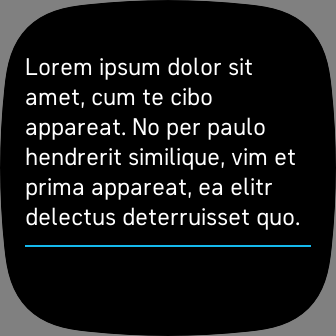## Arc

Draws an arc, circular or elliptical.

Attributes

• `arc-width`: arc thickness in pixels, or `%-expression`. Maximum 31.
• `start-angle`: start angle in degrees, with 0 at 12 o'clock.
• `sweep-angle`: length of the arc in degrees.
``````<svg viewport-fill="lightblue">
<arc x="25" y="50" width="100" height="100" fill="black"
arc-width="10" start-angle="0" sweep-angle="90" />
<arc x="215" y="50" width="100" height="100" fill="blue"
arc-width="10" start-angle="90" sweep-angle="90" />
<arc x="25" y="180" width="100" height="100" fill="magenta"
arc-width="10" start-angle="90" sweep-angle="180" />
<arc x="215" y="180" width="100" height="100" fill="red"
arc-width="10" start-angle="0" sweep-angle="360" />
</svg>
``````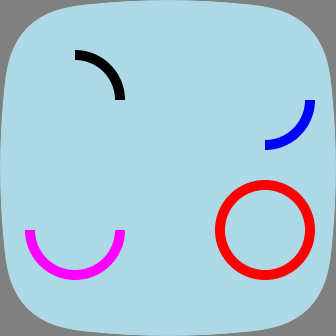## Defs

`<defs>` is a container element that should be used to include `<symbol>` and stylesheet definitions that will be used by the document.

Elements placed inside the `<defs>` container are not rendered.

Attributes

The defs element has no attributes.

## Section

This element is a container that can be used instead of the `<svg>` when only position and size properties of the container are required. It establishes a new coordinate system for the element it contains.

The `<section>` element has less attributes than `<svg>` and, therefore, consumes less memory.

Attributes

• `x`, `y`: position of the left, top-most corner.
• `width`, `height`: specify the size of the element.
``````<svg viewport-fill="green">
<section x="50%-50" y="50%-50" width="100" height="100">
<rect width="100%" height="100%" fill="red" />
</section>
</svg>
``````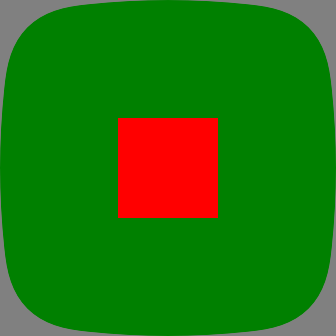## G Container

The `<g>` container can be used to translate (move), rotate, and/or scale, all of the elements it contains.

The transformations are applied to the coordinates of its child elements.

The `<animateTransform>` can be used to animate the `<g>` transformations. It is the only element that supports rotation and the only one that can be a valid parent of `<animateTransform>`.

### Translate (move)

``````<svg viewport-fill="lightblue">
<g transform="translate(50,100)">
<rect x="0" y="0" width="100" height="25" fill="blue" />
</g>
</svg>
``````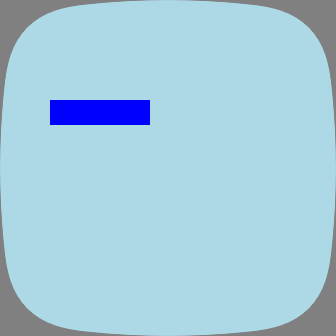### Rotate

``````<svg viewport-fill="lightblue">
<g transform="rotate(45)">
<rect x="50%-50" y="50" width="100" height="20" fill="blue" />
</g>
</svg>
``````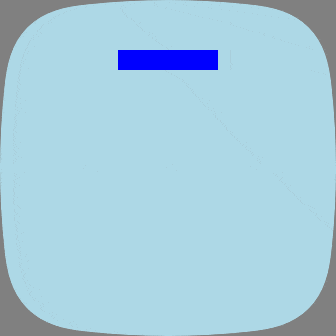### Scale

``````<svg viewport-fill="lightblue">
<g transform="scale(2.3)">
<rect x="10%" y="50" width="100" height="20" fill="blue" />
</g>
</svg>
``````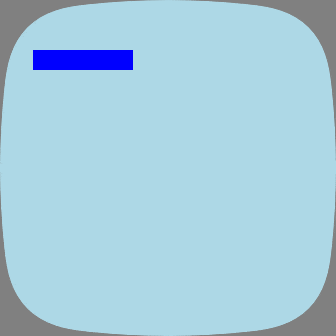The syntax `scale(factor)` implies uniform scaling: all coordinates are scaled by the same amount. Non-uniform scaling is done when individual scaling factors are specified: `scale(x,y,z)`. Use 1 to prevent scaling.

``````<svg viewport-fill="lightblue">
<g transform="scale(3,2.5,1)">
<rect x="30" y="50" width="50" height="20" fill="blue" />
</g>
</svg>
``````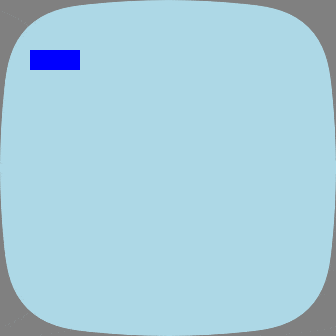The `<gradientRect>` element fills a rectangle with a color texture, without the use of a bitmap. It can blend two colors when the gradient type is `linear` or `radial`, or four colors when the type is `bilinear`.

Attributes

• `gradient-x1`, `gradient-y1`: define the first set of coordinates.
• `gradient-x2`, `gradient-y2`: define the second set of coordinates.
• `gradient-type`: the type of effect to use, `linear`, `radial`, or `bilinear`.
• `gradient-color1`, `gradient-color2`: specify colors that are used in all gradient types.
• `gradient-color3`, `gradient-color4`: specify colors that are only used in `bilinear` gradients.
• `gradient-opacity[1-4]`: each gradient color can also have opacity.

Note that for `linear` and `radial` gradients, `gradient-x1` must be less than or equal to `gradient-x2` and, similarly, `gradient-y1 <= gradient-y2`. `bilinear` gradients require that `gradient-y1 < gradient-y2` and `gradient-y1 < gradient-y2`.

``````<svg>
</svg>
``````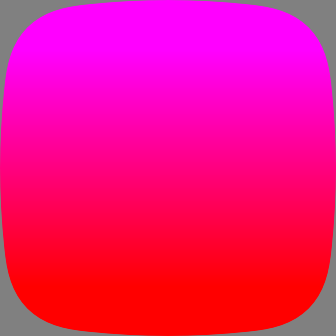``````<svg>
</svg>
``````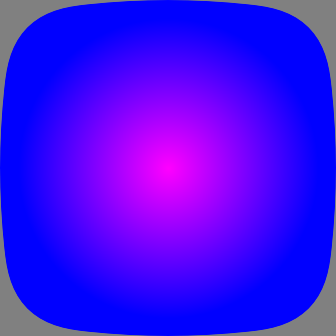``````<svg>
</svg>
``````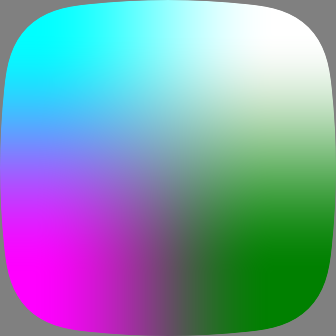`<mask>` is a container element that creates a mask which can be used by `<svg>` elements for visual effects. It can contain any graphical elements or container elements such as `<g>`.

The `<svg>` element that uses the mask element will be painted through the mask. Only the overlapping areas will remain.

In the following example, we define a `<mask>` with 4 rectangles, then use the mask by setting the `mask` attribute to the `#id` of the mask. Only the masked areas of the `<gradientRect>` will be visible.

``````<svg viewport-fill="#222">
<rect x="50%+15" y="50%"  width="60" height="10" />
<rect x="\$-160"  y="\$-10" width="60" height="10" />
<rect x="\$+15"   y="\$-85" width="10" height="60" />
<rect x="\$-10"   y="\$+40" width="10" height="60" />
</svg>
</svg>
``````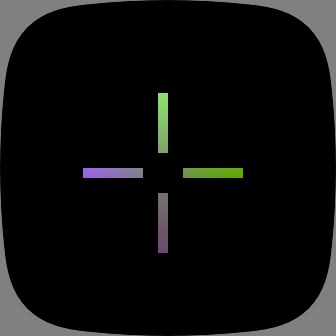## Template Symbols

By defining an element as a `<symbol>`, developers can reuse that same element multiple times without having to duplicate the same markup. The `<set>` element can be used to modify attributes of each instance of the `<symbol>`.

First we need to define our reusable symbol. We'll create 2 `<line>` elements inside our `<symbol>`:

``````<svg width="348" height="250" viewport-fill="lightblue">
<defs>
<symbol id="lines">
<line id="vertical" x1="50%" x2="50%" y1="0" y2="100%" fill="inherit" />
<line id="horizontal" x1="0" x2="100%" y1="50%" y2="50%" fill="inherit" />
</symbol>
</defs>
</svg>
``````

Then in order to use our symbol, we just need to `<use>` it as follows:

``````<use href="#lines" fill="black" />
<use href="#lines" x="50%-10" y="50%-10" fill="red" width="50" height="50" />
``````

If we want to modify any of the attributes, we can specify them with `<set>`:

``````<use href="#lines">
<set href="vertical" attributeName="fill" to="blue" />
<set href="horizontal" attributeName="fill" to="red" />
</use>
``````